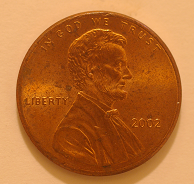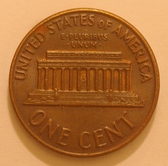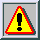The Lincoln CentA science project about the Lincoln Cent? Sure. After all, science is all around us in all aspects of our lives. One great benefit of this experiment is that the cost is literally pennies !! Before we begin, the coin is technically called a cent. Look at the reverse side ( the 'tails' side).It doesn't say penny does it? However, the word 'penny' is so popular, we will use it for the rest of this discussion.

S I Z E

 The diameter of the penny can be easily obtained by using a ruler. We measured it as .75 inches or 19 Millimeters. (Don't take our word for it though - try these measurements yourself.) Now determining the thickness is somewhat tougher isn't it? Instead of trying to measure 1 penny's thickness why not several stacked together? (We measured 30 pennies as 43 millimeters, making each penny 1.43 mm thick). A more precise method would be to use something not as common as a ruler - a micrometer. We used one and obtained a value of 1.27 mm (or .05 inches) for the thickness.

Now we can get a rough idea of its volume. The penny is a cylinder with an extremely low height. As with all cylinders, the formula is:

VOLUME = Pi * (Radius)2 * height

VOLUME = 3.14159 * 9.5 * 9.5 * 1.27

VOLUME = 360 cubic millimeters = .360 cubic centimeters.

You can recheck these figures - or recheck your own calculations - by going to the cylinder calculator.

An even more precise way for measuring the volume would be to use a measuring cup, a graduated cylinder or anything else used for measuring volume.

> > > > > IMPORTANT < < < < <If you use a measuring cup, make sure it is NEVER going to be used with food!!Please, no experiments with any food related equipment.

Okay now what? Well, fill the cup (or whatever) to a certain point with water and drop in a penny to see the volume rise. Of course the volume change would be minuscule. So, like the ruler experiment, why not drop in a great deal of pennies (remembering exactly how many you used), see the volume difference, divide it by the number of pennies, and you have the volume! If the volume is in Fluid Ounces and you want it in cubic centimetrs, just use the Ultra Converter.

M A S S

 We want to keep this discussion relatively short and so we don't want to present a long lecture concerning the concepts of mass and weight. These 2 terms are sometimes used interchangeably, but scientists always make a distinction between the two. (It would be a good idea for you to research these concepts yourself - and the Internet has LOTS of information out there). Just to give you a rough idea about weight and mass - in space, astronauts are weightless but they are NOT massless.

So, what is the mass of a penny? Well, an interesting change took place in 1982. Prior to that year, pennies were 95% Copper and 5% zinc. After 1982 the composition became 97.6% zinc and 2.4% copper. (1982 pennies can be either one of these. Oh and in 1943 pennies were made of a steel & zinc combination but we won't concern ourselves with those). So, the point is that pre-1982 pennies will have a different mass than pennies made after 1982. So, how about 'weighing' (determining the mass) of some pennies.

First, we will have to construct a crude scale. One way is to hang a plastic disposable cup from a rubber band and attach it vertically to a ruler. (Again, don't just take our suggestions. Perhaps you could find your own method of 'weighing' the pennies). Now we must use a 'standard' mass and the best substance for that would be water. Fill the cup with 100 milliliters (cubic centimeters) of water. This has a mass of 100 grams. (Remember the point on the ruler the cup reaches). Now empty the cup of water and fill it up with pre-1982 pennies until the cup reaches the same point as when you had it filled with water. The number of pennies in the cup will have a mass of 100 grams. (In case you are wondering, the number of pre-1982 pennies that equal 100 grams is about 32.(So each of these pennies has a mass of 3.1 grams). (However, do the experiment yourself). Now do the same with pennies made after 1982. (This will be about 40 pennies and so each of these pennies has a mass of about 2.5 grams).

Okay, you have now determined the size and the mass of pennies. Now time to move on to density.

D E N S I T Y

Density is defined by the formula:

Density = Mass ÷ Volume

(Not to confuse you anymore, but a lot of people use specific gravity and density interchangeably. However, specific gravity refers to the density of a substance compared to water - grams per cubic centimeter. (Almost always, densities are expressed this way but it is important to know the difference).

You have determined that a penny's volume is .360 cubic centimeters and the mass is 3.1 grams (pre-1982) and 2.5 grams (after 1982). So the densities are:
D = 3.1/.360 = 8.6 grams/ cubic centimeter(pre-1982)
D = 2.5/.360 = 6.94 grams/cubic centimeter (after 1982)

Now is there any way to verify your findings? Sure. For all intents and purposes we could say that pre-1982 pennies are all copper and the ones after 1982 are all zinc. Now looking up the densities we find Copper = 8.96 and Zinc = 7.13. Pretty good agreement wouldn't you say?

O T H E R     P R O P E R T I E S

Get a pre-1982 penny and drop it on the floor or on concrete or on a table, etc (but DON'T start scratching any furniture in the process). Anyway, did you notice the distinctive 'pinging' sound a copper penny makes? Now drop a penny made after 1982. The sound is much 'duller' isn't it? Why don't you research about copper and zinc to find out why this is so?

The next property to investigate is dangerous and so we advise youDO NOT DO THIS EXPERIMENT YOURSELF !!!! ⚠⚠ POSSIBILITY OF SKIN BURNS & FIRE HAZARD !!!!! ⚠Have your teacher, instructor or professor try this. (They are used to working with high temperatures).

Melting Point of Copper = 1,083°Celsius (=1,981°Fahrenheit)
Melting Point of Zinc = 419.6°Celsius (=787°Fahrenheit)

As we can see from above, there is a huge difference between the melting point of zinc and copper. So much of a difference that a zinc penny can be melted in a Bunsen burner but a copper penny cannot. To try this, we recommend clamping the penny with an alligator clip and holding that with pliers or Vise Grips™. The zinc penny will easily melt in the Bunsen burner flame. The copper penny will certainly get hot, it will even start to glow but it WON'T melt. We were patient enough to be able to melt a zinc penny in a candle flame. (This takes a while, is very sooty and smelly so be forewarned).

QUICK FACT
A pre-1982 penny contains about 28,000,000,000,000,000,000,000 copper atoms.
(In scientific notation that's 2.8 x 1022atoms).

Good luck with the project !!!